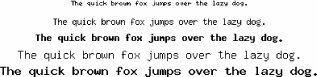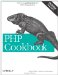# Recipe 17.5. Drawing Centered Text

#### 17.5.2. Problem

You want to draw text in the center of an image.

#### 17.5.3. Solution

Find the size of the image and the bounding box of the text. Using those coordinates, compute the correct spot to draw the text.

For built-in GD fonts, use the pc_ImageStringCenter( ) function, shown in Example 17-1.

##### pc_ImageStringCenter( )

 `function pc_ImageStringCenter(\$image, \$text, \$font) { // font sizes \$width = array(1 => 5, 6, 7, 8, 9); \$height = array(1 => 6, 8, 13, 15, 15); // find the size of the image \$xi = ImageSX(\$image); \$yi = ImageSY(\$image); // find the size of the text \$xr = \$width[\$font] * strlen(\$text); \$yr = \$height[\$font]; // compute centering \$x = intval((\$xi - \$xr) / 2); \$y = intval((\$yi - \$yr) / 2); return array(\$x, \$y); }`

For example:

`list(\$x, \$y) = pc_ImageStringCenter(\$image, \$text, \$font); ImageString(\$image, \$font, \$x, \$y, \$text, \$fore);`

For PostScript fonts, use the pc_ImagePSCenter( ) function, shown in Example 17-1.

##### pc_ImagePSCenter( )

 `function pc_ImagePSCenter(\$image, \$text, \$font, \$size, \$space = 0, \$tightness = 0, \$angle = 0) { // find the size of the image \$xi = ImageSX(\$image); \$yi = ImageSY(\$image); // find the size of the text list(\$xl, \$yl, \$xr, \$yr) = ImagePSBBox(\$text, \$font, \$size, \$space, \$tightness, \$angle); // compute centering \$x = intval((\$xi - \$xr) / 2); \$y = intval((\$yi + \$yr) / 2); return array(\$x, \$y); }`

For example:

`list(\$x, \$y) = pc_ImagePSCenter(\$image, \$text, \$font, \$size); ImagePSText(\$image, \$text, \$font, \$size, \$fore, \$back, \$x, \$y);`

For TrueType fonts, use the pc_ImageTTFCenter( ) function shown in Example 17-3.

##### pc_ImageTTFCenter( )

 `function pc_ImageTTFCenter(\$image, \$text, \$font, \$size) { // find the size of the image \$xi = ImageSX(\$image); \$yi = ImageSY(\$image); // find the size of the text \$box = ImageTTFBBox(\$size, \$angle, \$font, \$text); \$xr = abs(max(\$box, \$box)); \$yr = abs(max(\$box, \$box)); // compute centering \$x = intval((\$xi - \$xr) / 2); \$y = intval((\$yi + \$yr) / 2); return array(\$x, \$y); }`

For example:

`list(\$x, \$y) = pc_ImageTTFCenter(\$image, \$text, \$font, \$size); ImageTTFText(\$image, \$size, \$angle, \$x, \$y, \$fore, \$font, \$text);`

#### 17.5.4. Discussion

All three solution functions return the x and y coordinates for drawing. Of course, depending on font type, size, and settings, the method used to compute these coordinates differs.

For PostScript Type 1 fonts, pass pc_ImagePSCenter( ) an image allocated from ImageCreate( ) (or one of its friends) and a number of parameters to specify how to draw the text. The first three parameters are required: the text to be drawn, the font, and the font size. The next three are optional: the space in a font, the tightness between letters, and an angle for rotation in degrees.

Inside the function, use ImageSX( ) and ImageSY( ) to find the size of the canvas; they return the width and height of the graphic. Then call ImagePSBBox( ). It returns four integers: the x and y coordinates of the lower-leftmost location the text and the x and y coordinates of the upper-rightmost location. Because the coordinates are relative to the baseline of the text, it's typical for these not to be 0. For instance, a lowercase "g" hangs below the bottom of the rest of the letters; so in that case, the lower left y value is negative.

Armed with these six values, you can now calculate the correct centering values. Because coordinates of the canvas have (0,0) in the upper left corner, but ImagePSText( ) wants the lower left corner, the formula for finding \$x and \$y isn't the same. For \$x, take the difference between the size of the canvas and the text. This gives the amount of whitespace that surrounds the text. Then divide that number by two to find the number of pixels you should leave to the left of the text. For \$y, do the same, but add \$yi and \$yr. By adding these numbers, you can find the coordinate of the far side of the box, which is what is needed here because of the inverted way the y coordinate is entered in GD.

Intentionally ignore the lower left coordinates in making these calculations. Because the bulk of the text sits above the baseline, adding the descending pixels into the centering algorithm actually worsens the code; it appears offcenter to the eye.

To center text, put it together like this:

`function pc_ImagePSCenter(\$image, \$text, \$font, \$size, \$space = 0,                            \$tightness = 0, \$angle = 0) {     // find the size of the image     \$xi = ImageSX(\$image);     \$yi = ImageSY(\$image);     // find the size of the text     list(\$xl, \$yl, \$xr, \$yr) = ImagePSBBox(\$text, \$font, \$size,                                      \$space, \$tightness, \$angle);     // compute centering     \$x = intval((\$xi - \$xr) / 2);     \$y = intval((\$yi + \$yr) / 2);     return array(\$x, \$y); } \$image = ImageCreate(500,500); \$text = 'PHP Cookbook Rules!'; \$font = ImagePSLoadFont('/path/to/font.pfb'); \$size = 20; \$black = ImageColorAllocate(\$image, 0, 0, 0); \$white = ImageColorAllocate(\$image, 255, 255, 255); list(\$x, \$y) = pc_ImagePSCenter(\$image, \$text, \$font, \$size); ImagePSText(\$image, \$text, \$font, \$size, \$white, \$black, \$x, \$y); ImagePSFreeFont(\$font); header('Content-type: image/png'); ImagePng(\$image); ImageDestroy(\$image);`

Unfortunately, this example doesn't work for GD's built-in fonts or for TrueType fonts. There's no function to return the size of a string using the built-in fonts, and ImageTTFBBox( ) returns eight values instead of four. With a few modifications, however, you can accommodate these differences.

Because the built-in fonts are fixed width, you can easily measure the size of a character to create a function that returns the size of the text based on its length. Table 17-1 isn't 100 percent accurate, but it should return results within one or two pixels, which should be good enough for most cases.

##### Table 17-1. GD built-in font character sizes

Font number

Width

Height

1

5

6

2

6

8

3

7

13

4

8

15

5

9

15

Inside pc_ImageStringCenter( ), calculate the length of the string as an integral multiple based on its length; the height is just one character high. Note that ImageString( ) takes its y coordinate as the uppermost part of the text, so you should switch the sign back to a minus when you compute \$y.

Here is an example using all five fonts that centers text horizontally:

`\$text = 'The quick brown fox jumps over the lazy dog.'; for (\$font = 1, \$y = 5; \$font <= 5; \$font++, \$y += 20) {     list(\$x, \$y) = pc_ImageStringCenter(\$image, \$text, \$font);     ImageString(\$image, \$font, \$x, \$y, \$text, \$color); }`

The output is shown in Figure 17-8.

##### Centered GD built-in fontsFor TrueType fonts, you need to use ImageTTFBBox( ) or the more modern ImageFtBBox( ). (The function with TTF in the name is for FreeType version 1.x; the one with Ft is for FreeType 2.x.) It returns eight numbers: the (x,y) coordinates of the four corners of the text starting in the lower left and moving around counterclockwise. So the second two coordinates are for the lower right spot, and so on.

To make pc_ImageTTFCenter( ), begin with pc_ImagePSCenter( ) and swap this line:

`     // find the size of the text     list(\$xl, \$yl, \$xr, \$yr) = ImagePSBBox(\$text, \$font, \$size,                                      \$space, \$tightness, \$angle);`

with these:

`     // find the size of the text     \$box = ImageTTFBBox(\$size, \$angle, \$font, \$text);     \$xr = abs(max(\$box, \$box));     \$yr = abs(max(\$box, \$box));`

Here's an example of pc_ImageTTFCenter( ) in use:

`list(\$x, \$y) = pc_ImageTTFCenter(\$image, \$text, \$font, \$size); ImageTTFText(\$image, \$size, \$angle, \$x, \$y, \$white, \$black,              '/path/to/font.ttf', \$text);`

Recipe 17.4 for more on drawing text; Recipe 17.5 for more on centering text; documentation on ImageSX( ) at http://www.php.net/imagesx, ImageSY( ) at http://www.php.net/imagesy, ImagePSBBox( ) at http://www.php.net/imagepsbbox, ImageTTFBBox( ) at http://www.php.net/imagettfbbox, ImageFtBBox( ) at http://www.php.net/imageftbbox.PHP Cookbook: Solutions and Examples for PHP Programmers
ISBN: 0596101015
EAN: 2147483647
Year: 2006
Pages: 445

Similar book on Amazon